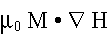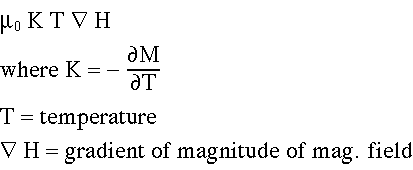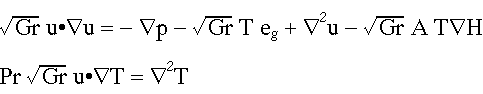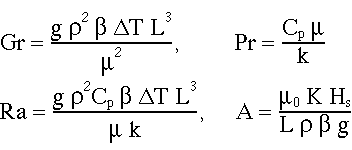Equations Governing Ferrofluid Flow and Heat Transfer

The equations are basically the Navier-Stokes equations augmented by a term representing the effect of the magnetic field. The full term isand the magnetism depends on the magnetic field and temperature. For a steady magnetic field in the absence of free surfaces the term involving temperature dependence becomesThe dimensionless form of the equations for a steady magnetic field iswhere the dimensionless groups are given by:These equations are solved in FIDAP using a special body force subroutine. Additional equations are used for the case of a rotating magnetic field (work in progress).

Main Page :::Heat Transfer in Box ::: Heat Transfer in 2D Model Transformer